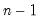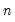Command Reference : Operator and Function Reference : Group Row Functions

Group Row Functions
These functions work on a group object. They may be used to create a new series where each row of that series is a function of the corresponding row of a group. Thus, if group G contains the series X, Y and Z, the @rmean function will return (X+Y+Z)/3.
These functions do not propagate NAs, i.e., they generate data based upon the observations within the group row that are non-NA.
As an example, the @rsum function will return a series where each row is equal to the sum of the non-NA elements in the row of a group.
Note that in the following descriptions, G is a named group in your workfile.

 Name Function Description @columns(g) number of columns. returns the number of columns in G. Note this is the same as “G.@count”, and will return the same number for every row. @rnas(g) row-wise number of NAs. the number of missing observations in the rows of G. @robs(g) row-wise number of non-NAs. the number of non-missing observations in the rows of G. @rvalcount(g,v) row-wise count of observations matching v. the number of observations with a value equal to v in the rows of G. Note v can be a scalar or a series @rsum(g) row-wise sum. the sum of the rows of G. @rmean(g) row-wise mean. the mean of the rows of G. @rmedian(g) row-wise median the median of the rows of G. @rprod(g) row-wise product the product of the rows of G. Beware overflow. @rquantile(g,q) row-wise quantile, where q is the quantile the quantile of the rows of G. The Cleveland quantile definition is used. @rstdev(g) row-wise standard deviation. the sample standard deviation of the rows of G. Note division is by. @rstdevp(g) row-wise population standard deviation. the population standard deviation of the rows of G. Note division is by. @rstdevs(g) row-wise sample standard deviation. the sample standard deviation of the rows of G. Note division is by, and this calculation is the same as @rstdev. @rvar(g) row-wise variance. the population variance of the rows of G. Note division is by. @rvarp(g) row-wise population variance the population variance of the rows of G. Note division is by, and this calculation is the same as @rvar. @rvars(g) row-wise sample variance. the sample variance of the rows of G. Note division is by. @rsumsq(g) row-wise sum-of-squares. the sum of the squares of the rows of G. @rfirst(g) row-wise first non-NA value. the value of the first non-missing observation in the rows of G. @rifirst(g) row-wise first non-NA index. the index (i.e., column number) of the first non-missing observation in the rows of G. @rilast(g) row-wise last non-NA index. the index (i.e., column number) of the last non-missing observation in the rows of G. @rimax(g) row-wise maximum index. the index (i.e., column number) of the maximum observation in the rows of G. In the case of ties, the first matching index is given. @rimin(g) row-wise minimum index. the index (i.e., column number) of the minimum observation in the rows of G. In the case of ties, the first matching index is given. @rlast(g) row-wise last non-NA value. the value of the last non-missing observation in the rows of G @rmax(g) row-wise maximum value. the value of the maximum observation in the rows of G. @rmin(g) row-wise minimum value. the value of the minimum observation in the rows of G.